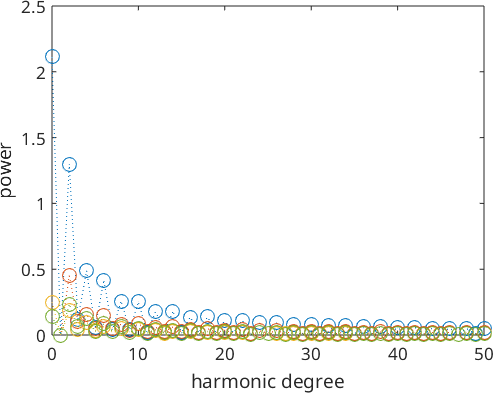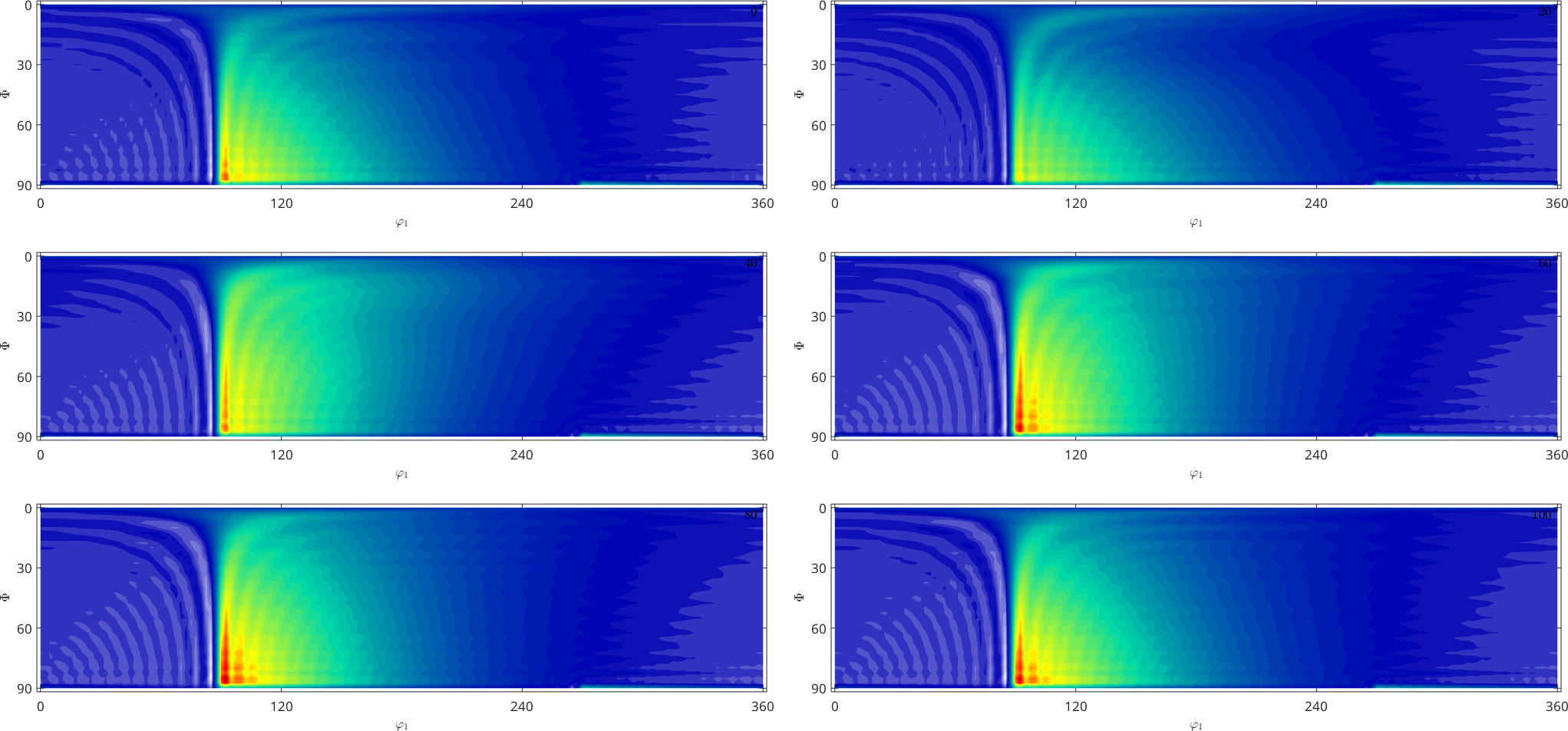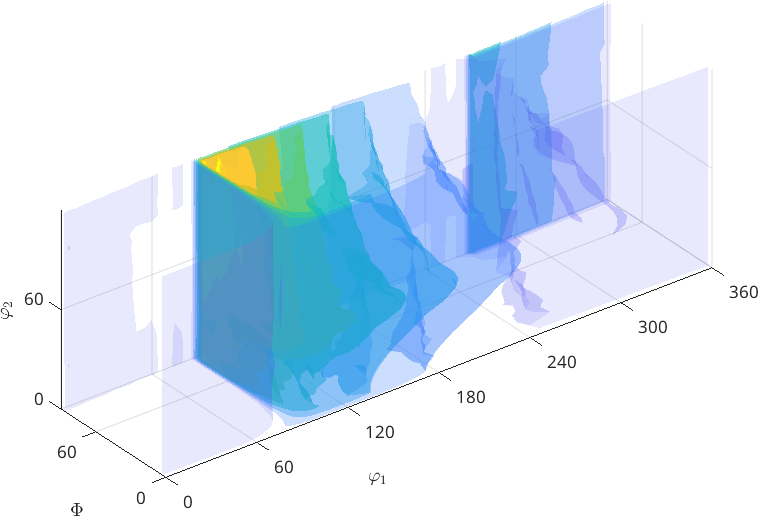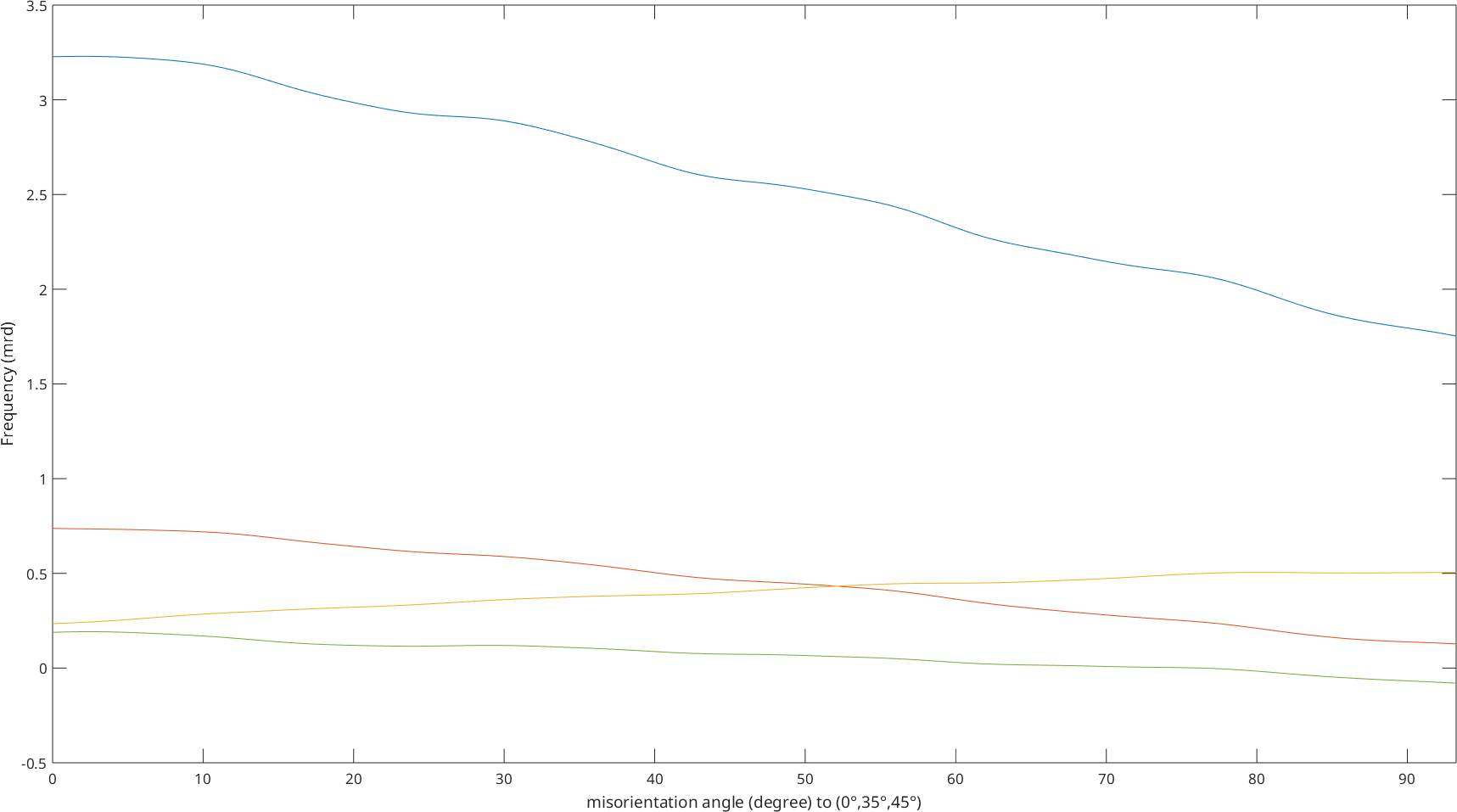Multivariate SO3Fun edit page

## Structural conventions of the input and output of multivariate SO3FunHarmonics

In this part we deal with multivariate functions of the form

$f\colon \mathcal{SO}(3) \to \mathbb R^n.$

• the structure of the nodes @rotations is always interpreted as a column vector
• the node index is the first dimension
• the dimensions of the SO3FunHarmonic itself is counted from the second dimension

For example we got four nodes $$R_1, R_2, R_3$$ and $$R_4$$ and six functions $$f_1, f_2, f_3, f_4, f_5$$ and $$f_6$$, which we want to store in a 3x2 array, then the following scheme applies to function evaluations:

$F(:, :, 1) = \pmatrix{f_1(v_1) & f_2(v_1) & f_3(v_1) \cr f_1(v_2) & f_2(v_2) & f_3(v_2) \cr f_1(v_3) & f_2(v_3) & f_3(v_3) \cr f_1(v_4) & f_2(v_4) & f_3(v_4)} \quad\mathrm{and}\quad F(:, :, 2) = \pmatrix{f_4(v_1) & f_5(v_1) & f_6(v_1) \cr f_4(v_2) & f_5(v_2) & f_6(v_2) \cr f_4(v_3) & f_5(v_3) & f_6(v_3) \cr f_4(v_4) & f_5(v_4) & f_6(v_4)}.$

For the intern Fourier-coefficient matrix the first dimension is reserved for the Fourier-coefficients of a single function; the dimensions of the functions itself begins again with the second dimension.

If $$\bf{\hat f}_1, \bf{\hat f}_2, \bf{\hat f}_3, \bf{\hat f}_4, \bf{\hat f}_5$$ and $$\bf{\hat f}_6$$ would be the column vectors of the Fourier-coefficients of the functions above, internally they would be stored in $$\hat F$$ as follows. $\hat F(:, :, 1) = \pmatrix{\bf{\hat f}_1 & \bf{\hat f}_2 & \bf{\hat f}_3} \quad\mathrm{and}\quad \hat F(:, :, 2) = \pmatrix{\bf{\hat f}_4 & \bf{\hat f}_5 & \bf{\hat f}_6}.$

## Defining a multivariate SO3FunHarmonic

Definition via function values

At first we need some vertices

Next we define function values for the vertices

Now the actual command to get a (2x1) SO3F1 of type $$~$$ SO3FunHarmonic is

It is also possible to interpolate one component by an SO3FunRBF, that means

This is only possible for univariate functions.

Definition via function handle

If we have a function handle for the function we could create a SO3FunHarmonic via quadrature. At first let us define a function handle which takes $$~$$ rotation as an argument and returns double:

Now we call the quadrature command to get (4x1) SO3F3 of type $$~$$ SO3FunHarmonic

Definition via Fourier-coefficients

If we already know the Fourier-coefficients, we can simply hand them in the format above to the constructor of SO3FunHarmonic.

## Operations which differ from an univariate SO3FunHarmonic

Some default matrix and vector operations

You can concatenate and refer to functions as Matlab does with vectors and matrices

You can conjugate the Fourier-coefficients and transpose/ctranspose the multivariate SO3FunHarmonic.

Some other operations

sum and mean

If we do not specify further options to sum or mean they give we the integral or the mean value back for each function. You could also calculate the conventional sum or the meanvalue over a dimension of a multivariate SO3FunHarmonic.

min/max

If the min or max command gets a multivariate SO3FunHarmonic the pointwise minimum or maximum is calculated along the first non-singelton dimension if not specified otherwise.

Therefore the function has to be real valued

Remark on the matrix product

At this point the matrix product is implemented pointwise and not as the usual matrix product.

## Visualization of multivariate SO3FunHarmonic

Similary to the univariate case we also can look at the Fourier coefficients of multivariate functions.The section plot and the 3d plot are performed only for the first component of a multivariate functionwhile the plot along a specific fibre includes all components.